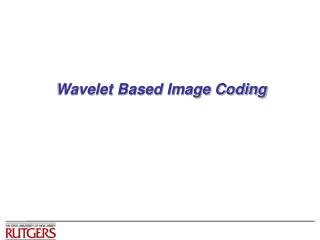DownloadDownload PresentationWavelet Based Image Coding

# Wavelet Based Image Coding

Télécharger la présentation## Wavelet Based Image Coding

- - - - - - - - - - - - - - - - - - - - - - - - - - - E N D - - - - - - - - - - - - - - - - - - - - - - - - - - -
##### Presentation Transcript

1. Wavelet Based Image Coding

2. power of 2 k = 2p + q – 1 “reminder” x 1 Construction of Haar functions • Unique decomposition of integer k (p, q) • k = 0, …, N-1 with N = 2n, 0 <= p <= n-1 • q = 0, 1 (for p=0); 1 <= q <= 2p (for p>0) e.g., k=0 k=1 k=2 k=3 k=4 … (0,0) (0,1) (1,1) (1,2) (2,1) … • hk(x) = h p,q(x) for x  [0,1]

3. Haar Transform • Haar transform H • Sample hk(x) at {m/N} • m = 0, …, N-1 • Real and orthogonal • Transition at each scale p is localized according to q • Basis images of 2-D (separable) Haar transform • Outer product of two basis vectors

4. Compare Basis Images of DCT and Haar See also: Jain’s Fig.5.2 pp136

5. x 1 Summary on Haar Transform • Two major sub-operations • Scaling captures info. at different frequencies • Translation captures info. at different locations • Can be represented by filtering and downsampling • Relatively poor energy compaction

6. Orthonormal Filters • Equiv. to projecting input signal to orthonormal basis • Energy preservation property • Convenient for quantizer design • MSE by transform domain quantizer is same as reconstruction MSE • Shortcomings: “coefficient expansion” • Linear filtering with N-element input & M-element filter  (N+M-1)-element output  (N+M)/2 after downsample • Length of output per stage grows ~ undesirable for compression • Solutions to coefficient expansion • Symmetrically extended input (circular convolution) &Symmetric filter

7. Solutions to Coefficient Expansion • Circular convolution in place of linear convolution • Periodic extension of input signal • Problem: artifacts by large discontinuity at borders • Symmetric extension of input • Reduce border artifacts (note the signal length doubled with symmetry) • Problem: output at each stage may not be symmetric From Usevitch (IEEE Sig.Proc. Mag. 9/01)

8. Solutions to Coefficient Expansion (cont’d) • Symmetric extension + symmetric filters • No coefficient expansion and little artifacts • Symmetric filter (or asymmetric filter) => “linear phase filters” (no phase distortion except by delays) • Problem • Only one set of linear phase filters for real FIR orthogonal wavelets  Haar filters: (1, 1) & (1,-1) do not give good energy compaction

9. Successive Wavelet/Subband Decomposition Successive lowpass/highpass filtering and downsampling • on different level: capture transitions of different frequency bands • on the same level: capture transitions at different locations Figure from Matlab Wavelet Toolbox Documentation

10. Examples of 1-D Wavelet Transform From Matlab Wavelet Toolbox Documentation

11. 2-D Example From Usevitch (IEEE Sig.Proc. Mag. 9/01)

12. Subband Coding Techniques • General coding approach • Allocate different bits for coeff. in different frequency bands • Encode different bands separately • Example: DCT-based JPEG and early wavelet coding • Some difference between subband coding and early wavelet coding ~ Choices of filters • Subband filters aims at (approx.) non-overlapping freq. response • Wavelet filters has interpretations in terms of basis and typically designed for certain smoothness constraints (=> will discuss more ) • Shortcomings of subband coding • Difficult to determine optimal bit allocation for low bit rate applications • Not easy to accommodate different bit rates with a single code stream • Difficult to encode at an exact target rate

13. Review: Filterbank & Multiresolution Analysis

14. Smoothness Conditions on Wavelet Filter • Ensure the low band coefficients obtained by recursive filtering can provide a smooth approximation of the original signal From M. Vetterli’s wavelet/filter-bank paper